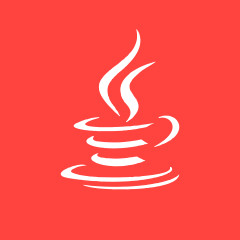### CAndroid C iOSC
61、请读程序: main() { int a=1,b=2; printf("%d\n",a=a+1,a+6,b+2);} 则上面程序的输出结果是____CC JAVA C++C
0基础求学习思路C
hi，大家。为啥开始int之后已经定义了一次i为0了，下面又要定义一次i为1？C C# C++C C++
m=6; y=2; y+=y-=m*=y; 运算过程和结果？？？ 求回答C
c语言，新手！CCCCCC JAVACC JAVA 数据结构C C++ GoC
sum=sum+i++等于多少CC C++ JAVACC C++ C#
C语言入门教程看完了。下一阶段看什么书或教程啊CCC JAVA 数据结构JAVA C JavaScript